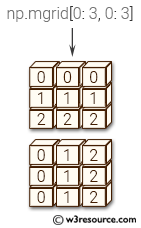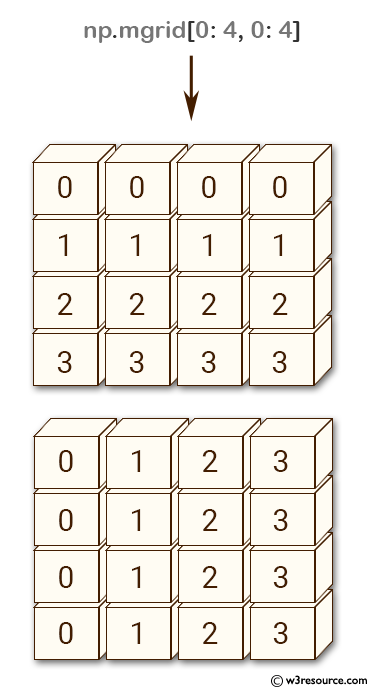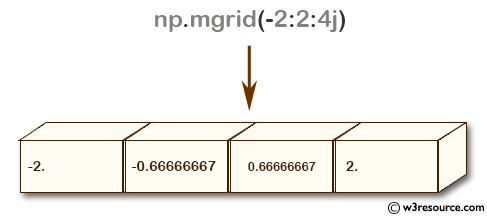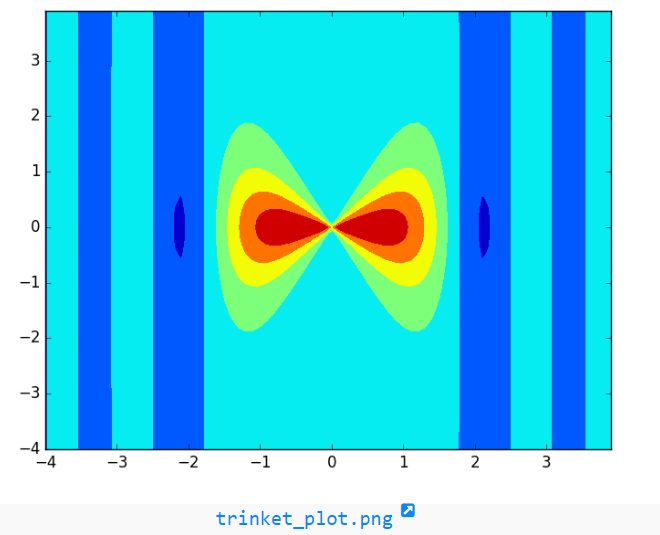# NumPy array creation: mgrid() function

## numpy.mgrid() function

The mgrid() function is used to get a dense multi-dimensional 'meshgrid'.
An instance of numpy.lib.index_tricks.nd_grid which returns an dense (or fleshed out) mesh-grid when indexed, so that each returned argument has the same shape. The dimensions and number of the output arrays are equal to the number of indexing dimensions. If the step length is not a complex number, then the stop is not inclusive.
However, if the step length is a complex number (e.g. 5j), then the integer part of its magnitude is interpreted as specifying the number of points to create between the start and stop values, where the stop value is inclusive.

Syntax:

`numpy.mgrid = <numpy.lib.index_tricks.nd_grid object>`Version: 1.15.0

Return value:

mesh-grid 'ndarrays' all of the same dimensions.

Example-1: NumPy.mgrid() function

``````>>> import numpy as np
>>> import numpy as np
>>> np.mgrid[0:4, 0:4]
array([[[0, 0, 0, 0],
[1, 1, 1, 1],
[2, 2, 2, 2],
[3, 3, 3, 3]],

[[0, 1, 2, 3],
[0, 1, 2, 3],
[0, 1, 2, 3],
[0, 1, 2, 3]]])
``````

Pictorial Presentation:Example-2: NumPy.mgrid() function

``````>>> import numpy as np
>>> np.mgrid[-2:2:4j]
array([-2.        , -0.66666667,  0.66666667,  2.        ])
``````

Pictorial Presentation:numpy.logspace.plot showPython - NumPy Code Editor:

Previous: meshgrid()
Next: ogrid()

﻿